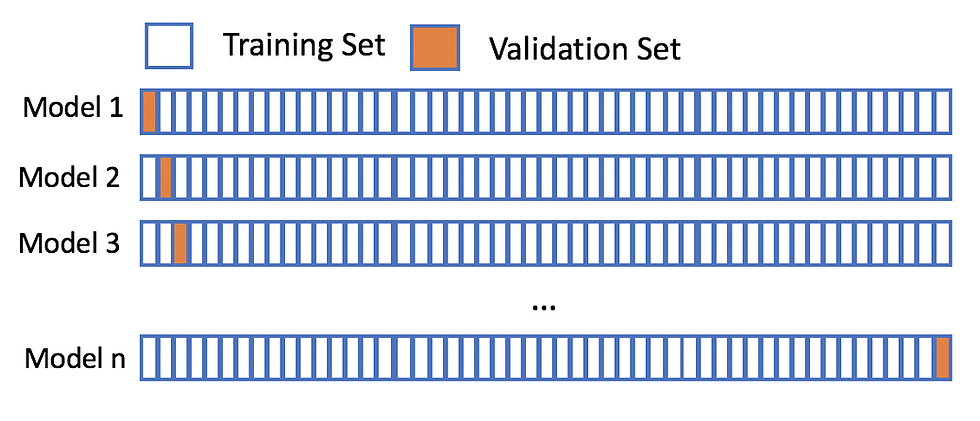top of pageSearch

# Cross-Validation TechniquesFor any model in machine learning it is considered as best practice if the model is tested with an independent data set. Usually, any model will work with an unknown data set which is also know as training set. In the real-life scenario, the model will be tested for the efficiency and the accuracy with a different and unique data set. In those circumstances we would want our model to be efficient enough or at least have the same efficiency as of the training data. This kind of testing is called cross-validation. Cross-validation is a statistical method used to estimate the skill of machine learning models.

Let me give you a simple example to make you all understand what exactly is cross-validation

Imagine you are trying to score a goal in an empty goal and it looks pretty easy to take number of goals from even a considerable distance. But the real test begins when you have a goal keeper and opponent team. Getting trained in a real match and still scoring goal is your best outcome.

## Types of Cross-Validation Technique

1. Leave one out cross validation

2. Leave P out cross validation

3. K-fold

4. Stratified K-fold

5. Holdout method

### Leave one out cross validation (LOOCV)

In this method each learning set is created by taking all the samples except one, the test set being the sample left out. Thus, for n samples, we have n different training sets and n different tests set. This cross-validation procedure does not waste much data as only one sample is removed from the training set.

If we have a data set with n observations then training data contains n-1 observation and test data contains 1 observation.### Leave P out

In this approach, p data points are left out of the training data. Let’s say there are m data points in the data set, then m-p points are used for training phase. The P data points are kept as a validation set. This is such an exhaustive technique because the above process that I have mentioned gets repeated for all the possible combination in the original data set and then the error is averaged for all trials, to give overall effectiveness. It becomes computationally infeasible since the model needs to train and validate for all the possible combinations.

### K-Fold method

In machine learning there is never enough data to train the model and even then, if we remove some part of the data there is always a risk of overfitting or it may not recognize the dominant pattern if not enough data is provided for training phase. By reducing the data, we also face the risk of low accuracy due to error induced by bias and to overcome this problem we need a method that will keep some data for training and some for testing. K-fold cross validation does that.

In this technique, the data is divided into k subsets. We take one subset from the date and treat it as the validation set for the model. We also keep K- 1 subset for training the model. The error estimation is averaged over all k trials to get total effectiveness of our model. The K subsets will be in the validation set at least once. It will also be included in the K-1 training set and this significantly reduces the error in the bias and also the variance.Typically, the value of K in K fold is 5 or 10 but it can take any value.

### Stratified K-fold

In this technique a slight change is made to the K-Fold cross-validation. The change is such that in each fold there will be approximately equal percentage of samples of the target class as the whole set, or in case of prediction problems the mean responsive value is approximately equal in all of the folds.image stack exchange

The above image is an example of binary classification problem where we want to predict if a passenger is male or female. We ensure that each fold has a percentage of passengers those are male and a percentage of passengers those are female.

### Hold out method

It is a simplified cross validation method. In hold out method we randomly assign data points to two datasets. The size is not relevant in this case because the basic idea behind this is to remove a part from your training set and use it to get prediction from the model from the model trained on rest of the data.